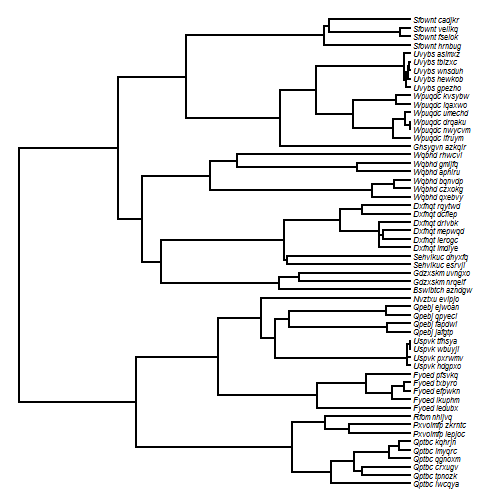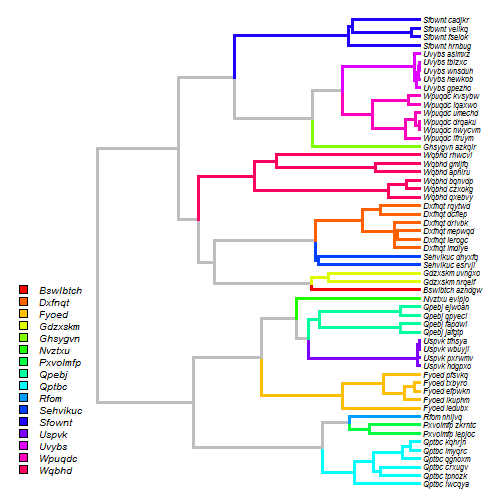## Saturday, December 24, 2016

### Using phytools to plot different genera with different colors

A phytools user asks the following:

“I am following your blog. I have a phylogenetic tree. I would like to color the tree according to their genus name. Could you please help me, providing the code in R?”

There are in fact many ways to plot a tree with edges or clades in different colors. I'm going to give a demo using `paintSubTree` and `plotSimmap` in phytools as follows.

First, let's imagine the following species-level tree in which I have used the syntax Genus_species for all taxon labels.

``````library(phytools)
species.tree
``````
``````##
## Phylogenetic tree with 56 tips and 55 internal nodes.
##
## Tip labels:
##  Rfom_nhijvq, Nvztxu_evipjo, Bswlbtch_azndgw, Ghsygvn_azkqlr, Qptbc_lwcqya, Qptbc_tpnozk, ...
##
## Rooted; includes branch lengths.
``````
``````plotTree(species.tree,ftype="i",fsize=0.7,color="black")
``````First, let's identify all the genera:

``````genera<-sapply(species.tree\$tip.label,function(x) strsplit(x,"_")[])
genera<-sort(unique(genera))
genera
``````
``````##   "Bswlbtch" "Dxfnqt"   "Fyoed"    "Gdzxskm"  "Ghsygvn"  "Nvztxu"
##   "Pxvolmfp" "Qpebj"    "Qptbc"    "Rfom"     "Sehvikuc" "Sfownt"
##  "Uspvk"    "Uvybs"    "Wpuqdc"   "Wqbhd"
``````

Next, find the MRCA of each genus. In the event that the genus contains only one member, we can just paint the terminal edge:

``````for(i in 1:length(genera)){
ii<-grep(genera[i],species.tree\$tip.label)
ca<-if(length(ii)>1)
findMRCA(species.tree,species.tree\$tip.label[ii]) else ii
species.tree<-paintSubTree(species.tree,ca,state=as.character(i),
anc.state="0",stem=TRUE)
}
``````

The following is a trick to remove map segments of zero length:

``````tol<-max(nodeHeights(species.tree))*1e-12
species.tree\$maps<-lapply(species.tree\$maps, function(x,tol)
if(length(x)>1) x[-which(x<tol)] else x,tol=tol)
``````

Now we can set our colors & plot them:

``````cols<-setNames(c("grey",rainbow(length(genera))),
0:length(genera))
plot(species.tree,fsize=0.7,colors=cols,ftype="i",split.vertical=TRUE,
lwd=3,xlim=c(-24,120))
par(font=3)
prompt=FALSE,x=-24,y=24)
``````That's basically the idea.

The tree for this example is obviously simulated. The following is the code that was used for simulation (taken from a previous post, here)::

``````library(phytools)
## first let's simulate our genus tree:
foo<-function() paste(sample(LETTERS,1),paste(sample(letters,
round(runif(1,min=3,max=7))),collapse=""),sep="")
genera<-replicate(16,foo())
genus.tree<-pbtree(n=length(genera),tip.label=genera,scale=80)
genus.tree\$edge.length[which(genus.tree\$edge[,2]<=Ntip(genus.tree))]<-
genus.tree\$edge.length[which(genus.tree\$edge[,2]<=Ntip(genus.tree))]+20
tips<-c()
for(i in 1:Ntip(genus.tree)){
n.genus<-sample(1:6,1)
if(n.genus>0) for(j in 1:n.genus)
tips<-c(tips,paste(genus.tree\$tip.label[i],
paste(sample(letters,6),collapse=""),sep="_"))
}